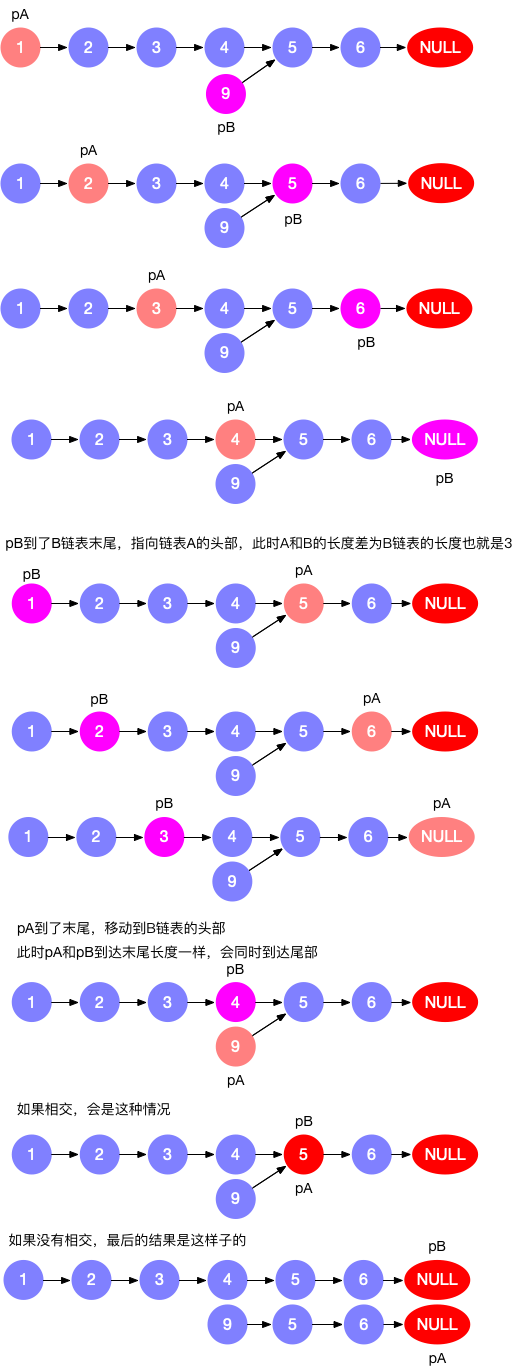# 日刷leetcode--简单版（五）### 119. 杨辉三角 II

#### 题目描述#### 解题思路

• 此题与118类似，直接冲118中返回最后一个数组即可，但是要优化到O(k)就显得不是那么容易了
• 公式:• 简单的来说就是前面的数乘以一个分数,这个分数从左到右分别为n/1, (n-1)/2, ..., 2/(n-1), 1/n，比如第3行就是分别乘以3/1，2/2，1/3
• 这里要注意的是是第0行，而非第一行
##### 示例代码
``````func getRow(rowIndex int) []int {
arr := make([]int,rowIndex+1)
if rowIndex == 0{
return arr
}
arr = 1
for i:= 1; i <= rowIndex; i++ {
arr[i] = arr[i-1] * (rowIndex-i+1)/i
}
return arr
}
``````

### 121. 买卖股票的最佳时机

#### 题目描述#### 解题思路1

• 双循环，对于每组 i 和 j（其中 j > i）我们需要找出 max(prices[j] - prices[i])

#### 解题思路二

• 循环一次，分别记录最小买入与最大利润即可
##### 示例代码
``````func maxProfit(prices []int) int {
maxPrices, min := 0, math.MaxUint32
for i := 0; i < len(prices); i++ {
if prices[i] < min {
min = prices[i] // 最小买入
}
if prices[i] - min > maxPrices {
maxPrices = prices[i] - min  // 最大利润
}
}
return maxPrices
}
``````

### 122. 买卖股票的最佳时机 II

#### 题目描述#### 解题思路

• 此题与121不同，121只需求出一段最高与最低即可，而此题需要求出多段
• 所谓利润，就是卖出比买入高，这就是利润，在此题中所映射出来的就是 `prices[i+1] > prices[i]`，这里我们假设今日卖出后，可在今日购买，这样一有利润我们就记录，这样就可以求出最高利润
• 举个栗子，比如数组是[1,2,5]，那么第一次判断发现 `2>1`，所以我们立即卖出得到利润 `2-1=1`，第二天判断 `5>2`，得到利润 `5-2=3`，所以总利润为 `1+3=4`;再举一个栗子[1,2,5,4,7]，这里我们可以得到 `2>1,5>2,7>4`,分别相减后相加得到总利润为 `7`
##### 示例代码
``````func maxProfit1(prices []int) int {
max := 0
for i := 0; i < len(prices) - 1; i++ {
if prices[i] < prices[i+1] {
max += prices[i+1] - prices[i]
}
}
return max
}
``````

### 125. 验证回文串

#### 题目描述#### 解题思路

• 一次循环，分别指定头指针与为指针，遇到非字母与数字则跳过
• 可在判断是否相同时进行大小写转换，也可在一开始就统一大小写
##### 示例代码
``````func isPalindrome(s string) bool {
for i, j := 0, len(s)-1; i < j; {
for (s[i] < 48 ||  (s[i] > 57 && s[i]< 65)  || (s[i] < 97 && s[i] > 90) || s[i] > 122) && i<j {
i++
}
for (s[j] < 48 ||  (s[j] > 57 && s[j]< 65)  || (s[j] < 97 && s[j] > 90) || s[j] > 122)  && i<j {
j--
}
if ToUpper(s[i]) != ToUpper(s[j]) {
return false
}
i++
j--
}
return true
}

func ToUpper(ascii uint8) uint8 {
if ascii >96 && ascii <= 122 {
ascii-=32
}
return ascii
}
``````

### 136. 只出现一次的数字

#### 题目描述#### 解题思路1

• 哈希表遍历，取出只出现一次的即可
##### 示例代码
``````func singleNumber(nums []int) int {
list := make(map[int]int, len(nums))
for i := 0; i < len(nums); i++ {
list[nums[i]] ++
}
for k,v := range list {
if v == 1{
return k
}
}
return 0
}
``````

#### 解题思路2

• ^作二元运算符就是异或，相同为0，不相同为1
• 解释一下异或，`1^1=0`,`0^2=2`
##### 示例代码
``````func singleNumbers(nums []int) int {
a := 0
for _,v :=range nums {
a ^= v
}
return a
}
``````

### 141. 环形链表

#### 题目描述#### 生成环链表

``````func (head *ListNode) CreateCycle(pos int) {
i := 0
if i == pos {
}
i++
}
}
``````

#### 解题思路--哈希

• 题目本身并不难，但是把这个题目看懂颇费心思
• 如示例1所示，遍历其生成的链表为 `3 -> 2 -> 0 -> -4 -> 2 -> 0 -> ....`
• 遍历单链表，如果当前节点为尾结点，这表示此链表不是环列表。如果当前节点存在哈希表中，这返回true,不存在则添加至哈希表中
• 时间复杂度O(n)，空间复杂度O(n)
##### 示例代码
``````func hasCycle(head *ListNode) bool {
nodes := make(map[*ListNode]int)
return  true
}else {
}
}
return false
}
``````

#### 解题思路--双指针

• 快慢指针同时遍历，当快指针指向链表尾部表示为非环链表
• 当快指针追上慢指针则表示当前为环链表
• 如同两个运动员赛跑，跑的快的运动员超过慢的运动员一圈的时候就可以判断他们跑的是环形跑道，如果跑的快的直接冲线了，那么他就不是环形跑道。
• 时间复杂度O(n)，空间复杂度O(n)
##### 示例代码
``````func hasCycle(head *ListNode) bool {
return false
}
for slow != fast {
if fast == nil || fast.Next == nil {
return false
}
slow = slow.Next
fast = fast.Next.Next
}
return true
}
``````

### 160. 相交联表

#### 题目描述#### 解题思路

• 思路有三种，暴力，哈希，和双指针，暴力和哈希比较简单，我采用了双指针的方式
• 指针 pA 指向 A 链表，指针 pB 指向 B 链表，依次往后遍历
• 如果 pA 到了末尾，则 pA = headB 继续遍历
• 如果 pB 到了末尾，则 pB = headA 继续遍历
• 图解如下##### 示例代码
``````func getIntersectionNode(headA, headB *ListNode) *ListNode {
return nil
}
for Pa != Pb {
if Pa == nil {
continue
}
if Pb == nil {
}
Pa = Pa.Next
Pb = Pb.Next
}
return Pa
}
``````